# Numerical analysis of helically finned tubes

Numerical analysis of helically finned tubes

Narmin B. Hushmandi

Department of Mechanical Engineering, Urmia University of Technology, Urmia P. O. Box 57155-419, Iran

Corresponding Author Email:
narminb@gmail.com
Page:
26-32
|
DOI:
https://doi.org/10.18280/psees.020105
12 May 2018
|
Accepted:
20 May 2018
|
Published:
31 March 2018
| Citation

OPEN ACCESS

Abstract:

Numerical analysis was done in this study to predict the behaviour of turbulent flow inside enhanced tubes. The tubes had helically inserted fins along the domain at multiple starts. Two different enhanced pipes at high and micro fin categories together with a smooth pipe were investigated numerically. Validations were done against existing experimental works published in open literature.

The RANS equations together with Realizable k-epsilon turbulence modelling were used. The tubes were horizontal with accounting for buoyancy effects along one coordinate perpendicular to the main flow. The open source CFD tool, OpenFOAM was used for computations. Validations showed fair agreement compared to measured data. Improvement of the heat transfer was assessed by comparison of the Nusselt numbers and friction factors with smooth pipe data and with acknowledged correlations.

Keywords:

CFD, heat transfer, finned tubes, geothermal energy

1. Introduction

It is well known that turbulent flow could cause higher heat transfer rates compared to laminar flow. Several design techniques exist to enhance the turbulence of internal flow inside pipes used in heat exchanger applications. These techniques include inlet disturbances within pipes, twisted tapes or coil inserts, dimpled or corrugated tubes, helically finned tubes at single or multiple number of starts. These designs increase the secondary flows, sweeps away the laminar flow close to the walls, increase the swirl and rotation within the flow and thus increases the heat transfer rates. Whatever method is used to increase the turbulence, the drawback is that the friction factor and the pressure drops are affected considerably. A compromise should be made between the amount of heat transfer gained and the resulting pressure drop.

Heat transfer in pipes and channels of various cross sections are used in many engineering applications such as chemical, power, energy and air supply systems. Both heating and cooling of fluids are common, and the flow can be laminar or turbulent as well as in single-phase or multiphase. Enhanced, or augmented, geometries are often used to increase the heat transfer rate for improved performance, higher energy gain or better design. By using enhanced pipes in a heat exchanger savings can be made in terms of operating and material costs.

The largest thermal resistance in a pipe or channel is close to the wall and the laminar boundary layer, therefore many techniques are used for enhancing the convective heat transfer related to breaking up the laminar boundary layer and promoting turbulence near the walls. Increasing the heat transfer area, with internal fins by example, leads to a higher heat transfer rate. Disruption of the laminar sublayer and boundary layer growth as well as boundary layer separation is positive for the heat transfer. Secondary flows and reattachment is also something that should be considered in heat transfer design. Features such as rotation, swirl and shedding lead to more turbulence which is a well known heat transfer promoter and increases the mixing of the fluid.

Common techniques to achieve these types of heat transfer improvements are straight, transversal or angled ribs, corrugated or dimpled tubes, twisted tapes and coil inserts. All techniques can be seen to increase the wall roughness promoting turbulence. The heat transfer area is often larger and swirling motion increases heat transfer for twisted tape inserts and ribbed fins.

The most important factor to investigate the heat transfer performance of a fluid flow through a pipe or channel is the Nusselt number which describes the convective to conductive heat transfer. All heat transfer increasing techniques will induce a higher flow resistance, or friction factor. The increased friction leads to a higher pressure drop and energy loss. A commonly used expression for investigated enhanced pipes is the efficiency expressed as the Nusselt to friction factor ratio divided by the same ratio for its corresponding smooth pipe.

The heat transfer of a smooth pipe can be expressed by several correlations developed for Nusselt number and friction factor, respectively. Dittus-Boelter or Gnielinski  are commonly used for Nusselt number predictions, and Blasius or Filonenko  are widely used for friction factor predictions. Ji, et al.  reviewed the experimental studies performed for single-phase heat transfer enhancement techniques of laminar and turbulent flows. They divided the techniques into four categories covering internal integral fins, twisted tape and coil inserts, corrugated tubes and dimpled or three-dimensional roughened tubes. They concluded that the enhancement ratio over the Dittus-Boelter correlation is between 2-4 for internally finned tubes, between 1.5-6 for twisted tape and coil inserts and 1.5-4 for corrugated and dimpled tubes. The increase in friction factor ratio is 1-4 over the fanning friction factor for internally finned tubes while between 2-13 for twisted tape and coil inserts and 2-6 and 3-5 for corrugated and dimpled tubes, respectively. They also found that internally finned tubes showed the best thermal hydraulic performance among others. Twisted tape and coil inserts showed good results for laminar flow, but the friction factor increased drastically in turbulent flow indicating restricted applicability for the technique.

Jensen and Vlakancic , experimentally investigated heat transfer inside helically finned pipes with varying number of starts, fin width, fin height and helix angles. Based on the friction factors two different type of pipes could be distinguished. The high finned pipes with less number of starts that had the same friction factor curve slope as the smooth pipe. The pipes with smaller fins and more number of starts, called micro-finned tubes, showed potential for higher Nusselt numbers than the high-finned pipes, but in the transitional lower Reynolds number region, the high finned pipes had higher Nusselt values, explained by the higher capacity for swirl motion.

In a numerical study by Xiaoyue and Jensen , the same variables as above was investigated. They found that both the friction factor and Nusselt number increased with number of starts and helix angle. Increased fin height yielded moderately higher friction factors and Nusselt numbers at lower helix angles but significantly higher at helix angles above 20 degrees. They concluded that the choice of fin width and the number of starts was important as the internal region between fins should not get too small. They also investigated fin tip profiles and found that for higher Reynolds numbers a rounded fin profile had lower friction factors, if the internal region between fins was large enough, while rectangular and triangular fins showed values similar to each other.

Meyer and Olivier  investigated the friction factor of enhanced pipes in the laminar to turbulent region experimentally. They found transition to occur earlier than the corresponding smooth pipes. A secondary transition was present between Reynolds numbers of 3000-10,000.

Kim et al.  investigated both micro-finned and high-finned tubes numerically. They showed the high performance of micro-finned tubes to a highly diffusive turbulent flow in connection with fins geometry rather than the increased heat transfer surface area. They also suggested that there are no differences in the governing heat transfer mechanism between a high finned tube and a micro-finned tube. Furthermore they showed the flow inside the internal region between the fins of micro-finned tubes to be predominantly laminar and the bulk turbulent flow not to be in contact with the surface, this tendency was thought to be connected to the surface resistance caused by the micro-fins. A k-epsilon model was used and was validated against the experimental data by Jensen and Vlakancic . Both a high-finned pipe and a micro-finned pipe were simulated and agreement of friction factor and Nusselt numbers where within 15% for Reynolds numbers above 18,000. They acknowledged that an isotropic turbulence model was not able to capture the complex flow phenomenon such as separations and re-laminarization, but the acceptable overall agreement with the experimental data justified the usage of the novel turbulence models.

Many experimental investigations of heat transfer and flow characteristics of internally finned tubes have been made over the years for flow in the laminar or fully turbulent region. Published investigations with numerical tools are limited but offers potential as the actual flow field can be described and analysed whereas the experimental investigations only deliver performance parameters. The current investigation covers internally finned pipes used in geothermal applications.

2. Geometry of the Tubes

Table 1 shows the details of the geometry of the computed smooth and helically finned tubes. One high fin and one micro fin tubes are selected for investigation.

Table 1. Geometry of computed smooth and internally finned tubes

 Tube Number Inside diameter Di (mm) Number of fins N Fin height e (mm) Fin thickness s (mm) Fin helix angle $\gamma$$\left(^{\circ}\right)$ H Group 1(smooth) 21.18 - - - - - - 2 (ref 1) 22.08 54 0.44 0.54 45 0.04 Micro 3 (ref 1) 23.78 14 1.2 1.02 30 0.1 High

3. Non-dimensional Parameters

Experimental measurement data of Jensen and Vlakanic  was used to validate the numerical computations presented in this paper. Fanning friction factor based on nominal internal diameter and reduced Nusselt numbers are used for the comparisons. The friction factor is computed according to Eq. 1:

$f=\frac{\Delta P \times D}{4 \times L \times \rho \times V^{2}}$    (1)

where $\Delta P$ is the difference between the area-weighted static pressure averages of two surfaces placed one complete turns apart at a fully developed region. L is the corresponding length of one complete turn. D is the nominal internal diameter of the pipe. $\rho$ is the fluid density and is assumed constant. V is the average velocity in the pipe section.

Nusselt numbers are computed according to Eq. 2 and are normalized with the Prandtl number at the average fluid temperature along the domain.

$N u=\frac{h \times D}{k}$    (2)

where h is the convective heat transfer coefficient and k is the thermal conductivity of the fluid. The type of the flow weather to be laminar, turbulent or in transition depends mainly on the Reynolds number.  Reynolds number is defined for the flow inside pipes according to Eq. 3:

$R e=\frac{V_{a v g} D}{v}=\frac{\rho V_{a v g} D}{\mu}$    (3)

It is common practice to assume the flow under Re=2300 inside circular pipes to be laminar and above Re=4000 to be fully turbulent.

4. Computational Methods

Due to the limitation in computational resources, this study is confined to turbulent flows. The hydrodynamic entry length for turbulent flow can be approximated according to Shah and Bhatti  as Eq. (4):

$L_{h, t u r b u l e n t}=1.359 . D . R e^{1 / 4}$    (4)

Since the Reynolds number studied in this paper is 10000, the longest entry length corresponding to Re=10000 is equal to about 13.6D according to Eq. (4). This length is much shorter than the length of computational grids in this study.

Linux Ubuntu 12.0.4 was used as operating system. Open source CFD Solver Open FOAM 2.3.0 and post processor ParaView 4.1.0 was used. Computations were done with parallel processing on a single PC of 16 GB RAM, 8 CPUs, 16 processors and 3.1 Hz CPU speed. A steady-state solver for buoyant, turbulent flow of incompressible fluids was employed in OpenFOAM. Open FOAM uses a finite volume based method. In the momentum equation, the pressure-velocity coupling was done using SIMPLE algorithm. Main flow was incompressible water with constant density. The governing equations were the Reynolds-averaged Navier Stokes equations and the Realizable k-epsilon turbulence modeling was used. Hexahedral cells in a structured manner were used to mesh the computational volume.

Longitudinal extent of the computational domain was chosen to be 20 complete turns. Thus the longitudinal extent for a pipe with a helix angle of 45 degrees equals to 20$\pi D$. Total number of volume cells were about 10 m cells in each computational case.

All of the tubes were taken horizontal with z-axis along the main flow direction. Gravity acted perpendicular to the main flow direction. The entrance Reynolds number was taken 10000 in all of the computational cases. One reason was to keep the flow in the turbulent region and to reach a fully developed flow within the computational grid and the other reason was that the friction factor and heat transfer data existed only above this Reynolds number. The y+ values were kept around 30. In the proceeding sections, each of the computed cases is presented separately and comparisons against the correlations or experimental data are performed.

Velocity at the inlet was uniform velocity corresponding to the specified Reynolds number, the pipe walls were no-slip condition. The entrance flow temperature was 273.16K and the pipe walls were at a constant temperature condition equal to 277.16K.

5. Tube No. 1 (Smooth Channel)

## 1.pngFigure 1. Details of the computational grid shown at a cross section and along the domain for tube number 1

Details of the computational grid at a cross section perpendicular to the longitudinal axis and along the main flow direction are presented in Fig. 1.

Figure 2 shows the contours of velocity component along the main flow direction (Vz) at plane cut through the centre of the pipe. Vz is normalized with the inlet velocity and plotted in longitudinal domain for tube number 1 at Re=10000. The length of the channel is normalized with the pipe total length.

## 2.pngFigure 2. Longitudinal velocity normalized with the inlet velocity for tube number 1 at a section passing the center

It can be seen from the figure above that a fully developed flow is reached after 20 percent of the channel length is passed. Similarly, profiles of temperature along the domain are plotted in Fig. 3 for smooth tube. Development of the temperature profile is seen along the channel.

## 3.png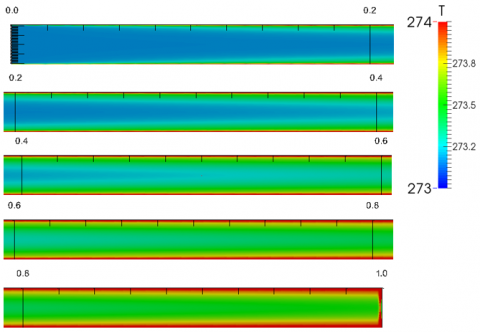Figure 3. Longitudinal temperature profile for tube number 1 at a cross section passing the center

Profiles of turbulent kinetic energy along the domain are plotted in Fig. 4 for smooth tube.

## 4.pngFigure 4. Longitudinal profile of turbulent kinetic energy for tube number 1 at a cross section passing the center

Figure 5 shows the Nusselt number on the outer walls of the smooth tube while the pipe diameter is taken as the characteristic length. The non-dimensional heat transfer coefficient is considerably higher at the entrance region.

## 5.pngFigure 5. Contours of Nusselt number along the domain on the outer walls of tube number 1

Two lines perpendicular to each other, one along the x-axis and the other along the y-axis are marked at the inlet and streamlines originating from them are plotted along the domain in Fig. 6.

## 6.png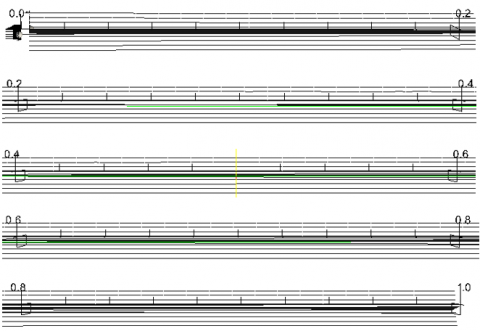Figure 6. Streamlines originating from two perpendicular lines at the inlet (x and y-axis) plotted along the domain for tube number 1

The computational results of smooth tube are compared to correlations of Gnielinski  and Filonenko . Friction factors are computed according to Eq. 1. ΔP is the area-averaged pressure difference between two cross sections at the fully developed region. Similarly, for the Nusselt number, an area-weighted average at the fully developed region on the outer walls are used.

Table 2. Numerical results of smooth tube compared with correlations by Gnielinski  and Filonenko 

 Computed Value Correlations Nusselt Number 110.64 87.1 Friction Factor 0.00486 0.00787

6. Tube No. 2 (N=54, Γ=45°)

Details of the computational grid at a cross section perpendicular to the longitudinal axis and along the main flow direction are presented in Fig. 7.

## 7.png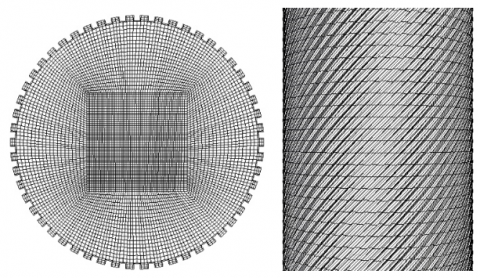Figure 7. Details of the computational grid shown at a cross section and along the domain for tube number 2

The velocity component along the main flow direction (Vz) is normalized with the inlet velocity and plotted along the domain at a cross section passing the centre for tube number 2 at Re=10000 in Fig. 8. The length is normalized with the pipe total length.

## 8.png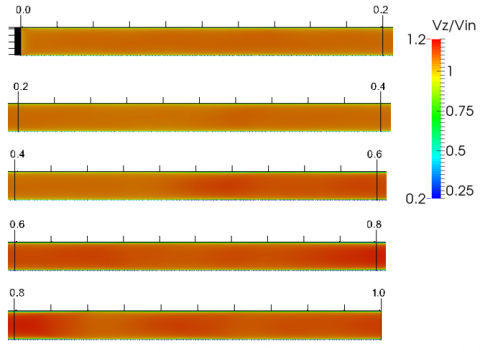Figure 8. Longitudinal velocity normalized with the inlet velocity for tube number 2 at a section passing the centre

Profiles of temperature along the domain are plotted in Fig. 9 for tube number 2.

## 9.png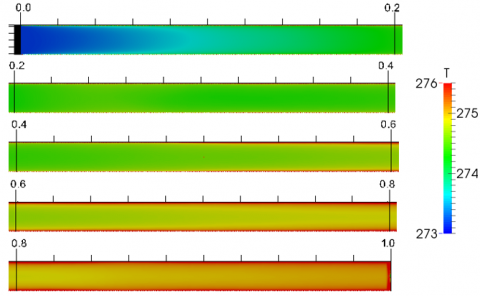Figure 9. Longitudinal temperature profile for tube number 2 at a cross section passing the center

Profiles of turbulent kinetic energy along the domain are plotted in Fig. 10. The turbulent kinetic energy has its local higher value at half of the pipe length.

## 10.png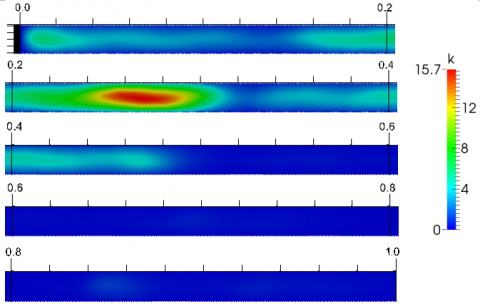Figure 10. Longitudinal profile of turbulent kinetic energy for tube number 2 at a cross section passing the center

Figure 11 shows the non-dimensional heat transfer coefficient (Nusselt number) on the outer walls of the tube. The Nusslet numbers are considerable higher compared to the corresponding smooth pipe.

## 11.png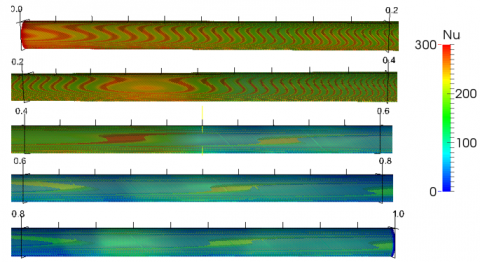Figure 11. Contours of Nusselt number along the domain on the outer walls of tube number 2

Two lines perpendicular to each other, one along the x-axis and the other along the y-axis are marked at the inlet and streamlines originating from them are plotted along the domain in Fig. 12.

## 12.png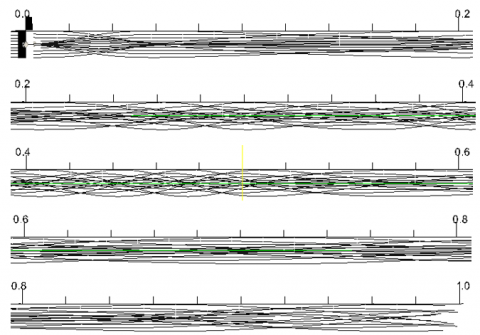Figure 12. Streamlines originating from two perpendicular lines at the inlet (x and y-axis) plotted along the domain for tube number 2

The computational results of smooth tube are compared to correlations of Gnielinski  and Filonenko . Friction factors are computed according to Eq. 1. ΔP is the area-averaged pressure difference between two cross sections at the fully developed region. Similarly, for the Nusselt number, an area-weighted average at the fully developed region on the outer walls are used.

Table 3. Numerical results of tube number 2 compared with experimental data of Jensen and Vlakanic, 

 Computed Value Experimental Nusselt Number 208.385 219 Friction Factor 0.0241 0.0144

A fair agreement is observed for the Nusselt number values. The difference in friction factor results is expected due to the coarse mesh near the wall regions.

7. Tube No. 3 (N=14, Γ=30°)

Details of the computational grid at a cross section perpendicular to the longitudinal axis and along the main flow direction are presented in Fig. 13.

## 13.png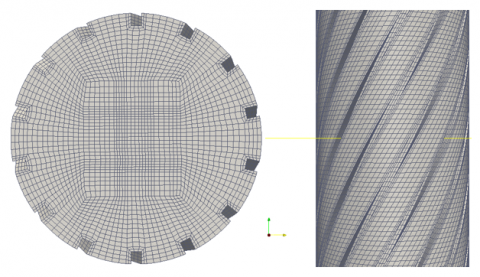Figure 13. Details of the computational grid shown at a cross section and along the domain for tube number 3

The velocity component along the main flow direction (Vz) is normalized with the inlet velocity and are plotted along the domain at a cross section passing the centre for tube number 3 at Re=10000 in Fig. 14. The length is normalized with the pipe total length.

## 14.pngFigure 14. Longitudinal velocity normalized with the inlet velocity for tube number 3 at a section passing the center

Profiles of temperature along the domain are plotted in Fig. 15.

## 15.pngFigure 15. Longitudinal temperature profile for tube number 3 at a cross section passing the center

Profiles of turbulent kinetic energy along the domain are plotted in Fig. 16 for tube number 3. Here the higher kinetic energy is seen up to 25 percent of the total length.

## 16.pngFigure 16. Longitudinal profile of turbulent kinetic energy for tube number 3 at a cross section passing the center

Figure 17 shows the non-dimensional heat transfer coefficient (Nusselt number) on the outer walls of the tube number 3. The observed local Nusselt numbers are considerably lower in this case compared to the low-fin pipe.

## 17.pngFigure 17. Contours of Nusselt number along the domain on the outer walls of tube number 3

Two lines perpendicular to each other, one along the x-axis and the other along the y-axis are marked at the inlet and streamlines originating from them are plotted along the domain in Fig. 18. Longitudinal coordinate is non-dimensionalzed with the total length of the channel.

## 18.png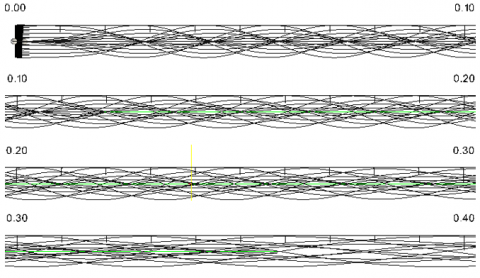Figure 18. Streamlines originating from two perpendicular lines at the inlet (x and y-axis) plotted along the domain for tube number 3

The computational results of tube number 3 are compared to experimental data by Jensen and Vlakanic, . Friction factors are computed according to Eq. 1. ΔP is the area-averaged pressure difference between two cross sections at the fully developed region. Similarly, for the Nusselt number, an area-weighted average at the fully developed region on the outer walls are used and the tube inside diameter is taken as the characteristic length.

Table 4. Numerical results of tube number 3 compared with correlations by experimental data of Jensen and Vlakanic, 

 Computed Value Experimental Nusselt Number 101 196 Friction Factor 0.0096 0.0174

Here, the computed average Nusselt numbers does not show very good agreement with the experimental results. The reason could be due to the coarse mesh used in the computations which did not allow to computer the laminar sub-layer profile correctly. Due to the less number of fins in the high-fin pipe, it is expected to have a laminar flow in the internal region between the fins and correctly modelling of the laminar sub-layer is crucial in obtaining a better agreement with the experimental results.

8. Conclusions

A numerical model was developed to simulate the flow and heat transfer fields in helically finned tubes used in geothermal applications. Two finned tubes corresponding to high and low fin types together with a smooth pipe were computed numerically. The chosen Reynolds number was 10000 to be in fully developed region and yet to be low enough to be suitable for geothermal applications. Twenty complete turns were computed in the longitudinal direction. Due to the computational limitations, the near wall y-plus values were kept at around 30. The computations showed that a fair agreement was seen between the numerically computed values and experimental data for the low-fin pipe but the disagreement was large for the high-fin case. Overall, the numerical simulations gave a very good view to the flow behaviour in such applications and insight to improvement of the design.

Nomenclature
 D diameter [mm] e Fin height [mm] h Convective heat transfer coefficient $\left[W m^{-2} K^{-1}\right]$ H non-dimensional fin height （= 2e/di ） [-] k Thermal conductivity of fluid $\left[W m^{-1} K^{-1}\right]$ L Length [m] N Number of fins p Static pressure [Pa] Re Reynolds number [-] s Fin thickness [mm] v velocity [m·s-1] Greek symbols $\gamma$ Fin helix angle [°] $\Delta$ Difference $\mu$ Dynamic viscosity [Pa·s-1] $\rho$ density [kg·m-3] $\tau$ Shear stress [Pa] $v$ Kinematic viscosity Subscripts avg Average h hydrodynamic i internal in inlet x, y, z Components in respective coordinate directions
References

 Cengel YA, Cimbala JM. (2006). Fluid Mechanics: Fundamental and Applications. The McGraw-Hill Companies. Inc.

 Filonenko GK. (1960). Hydraulischer wiederstand von rohrleitungen. Teploenergetika 1: 1098-1099.

 Gnielinski V. (1976). New equations for heat and mass transfer in turbulent pipe and channel flow. International Journal of Chemical Engineering 16: 359-368.

 Jensen MK, Vlakancic A. (1999). Technical note: Experimental investigation of turbulent heat transfer and fluid flow in internally finned tubes. International Journal of Heat and Mass Transfer 1343-1351.

 Ji W, Jacobi A, He Y, Tao W. (2015). Review: Summary and evaluation on single-phase heat transfer enhancement techniques of liquid laminar and turbulent pipe flow. International Journal of Heat and Mass Transfer 88735-754.

 Kim J, Jansen K, Jensen M. (2004). Analysis of heat transfer characteristics in internally finned tubes. Numerical Heat Transfer: Part A – Applications 46(1).

 Meyer JP, Olivier JA. (2011). Transitional flow inside enhanced tubes for fully developed and developing flow with different types of inlet disturbances: Part II-heat transfer. International Journal of Heat and Mass Transfer 54: 1598-1607.

 Shah RK, Bhatti MS. (1987). Laminar Convective Heat Transfer in ducts. Handbook of single-phase convective heat transfer, John Wiley & Sons, New York, pp. 3.1-3.137.

 Xiaoyue L, Jensen M. (2001). Geometry effects on turbulent flow and heat transfer in internally finned tubes. Journal of Heat Transfer 123(6).